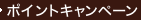•### Statistical Intervals : A Guide for Practitioners

• 提携先の海外書籍取次会社に在庫がございます。通常2週間で発送いたします。
重要ご説明事項
1. 納期遅延や、ご入手不能となる場合が若干ございます。
2. 複数冊ご注文の場合、分割発送となる場合がございます。
3. 美品のご指定は承りかねます。
• ≪洋書のご注文につきまして≫ 「海外取次在庫あり」および「国内仕入れ先からお取り寄せいたします」表示の商品でも、納期の目安期間内にお届けできないことがございます。あらかじめご了承ください。

• 製本 Hardcover:ハードカバー版／ページ数 392 p.
• 言語 ENG
• 商品コード 9780471887690
• DDC分類 519.5

### Full Description

Presents a detailed exposition of statistical intervals and emphasizes applications in industry. The discussion differentiates at an elementary level among different kinds of statistical intervals and gives instruction with numerous examples and simple math on how to construct such intervals from sample data. This includes confidence intervals to contain a population percentile, confidence intervals on probability of meeting specified threshold value, and prediction intervals to include observation in a future sample. Also has an appendix containing computer subroutines for nonparametric statistical intervals.

### Table of Contents

`  Overview of Different Types of Statistical  Intervals.  Constructing Statistical Intervals Assuming a  Normal Distribution Using Simple Tabulations.  Methods for Calculating Statistical Intervals  for a Normal Distribution.  Distribution-Free Statistical Intervals.  Statistical Intervals for Proportions and  Percentages (Binomial Distribution).  Statistical Intervals for the Number of  Occurrences (Poisson Distribution).  Sample Size Requirements for Confidence  Intervals on Population Parameters.  Sample Size Requirements for Tolerance  Intervals, Tolerance Bounds, and  Demonstration Tests.  Sample Size Requirements for Prediction  Intervals.  A Review of Other Statistical Intervals.  Other Methods for Setting Statistical  Intervals.  Case Studies.  Epilogue.  Appendices.  References.  Author Index.  Subject Index.`